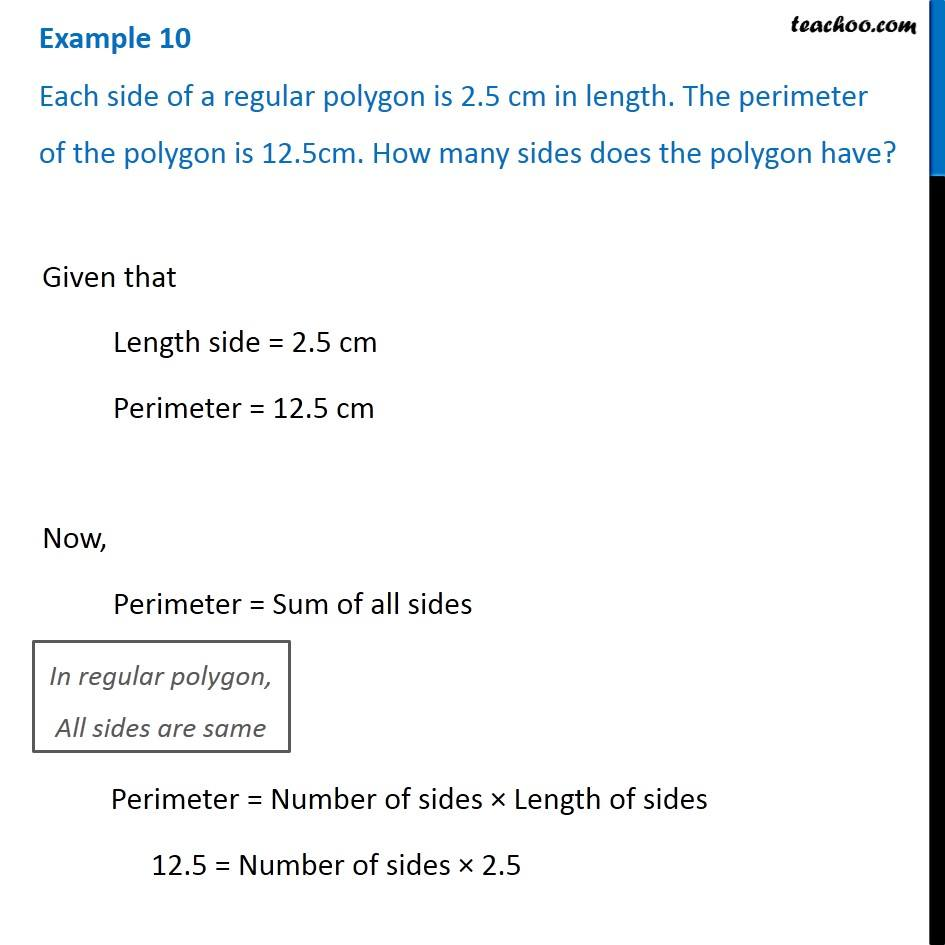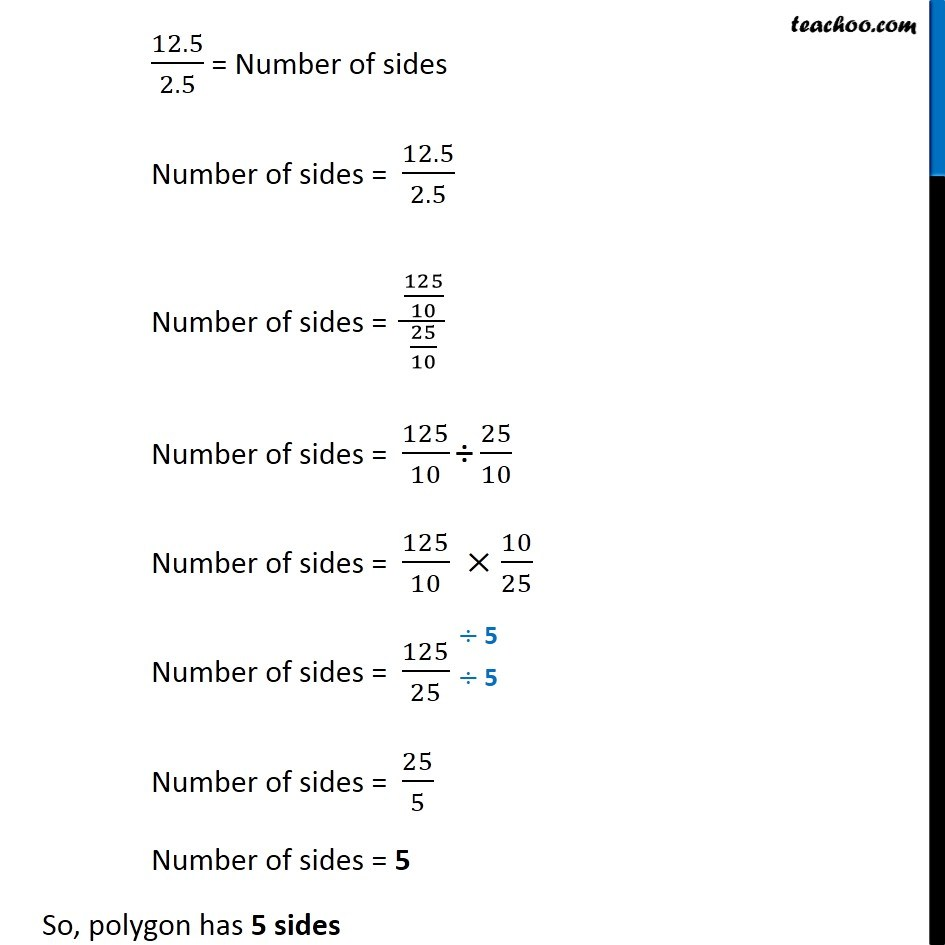Examples

Chapter 2 Class 7 Fractions and Decimals
Serial order wise### Transcript

Example 11 A car covers a distance of 89.1 km in 2.2 hours. What is the average distance covered by it in 1 hour?Distance covered in 22 hours = 89.1 km Distance covered in 1 hours = 89.1/2.2 km = 891/22 = 40.5 So, Distance covered by car in 1 hour = 40.5 km Example 10 Each side of a regular polygon is 2.5 cm in length. The perimeter of the polygon is 12.5cm. How many sides does the polygon have?Given that Length side = 2.5 cm Perimeter = 12.5 cm Now, Perimeter = Sum of all sides Perimeter = Number of sides × Length of sides 12.5 = Number of sides × 2.5 12.5/2.5 = Number of sides Number of sides = 12.5/2.5 Number of sides = (125/10)/(25/10) Number of sides = 125/10 "÷" 25/10 Number of sides = 125/10 ×10/25 Number of sides = 125/25 Number of sides = 25/5 Number of sides = 5 So, polygon has 5 sides